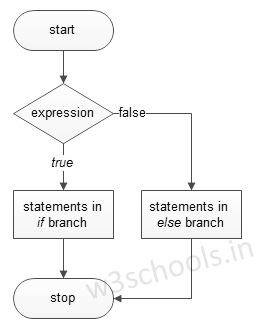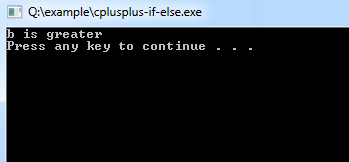# C++ if-else Statements

If else statements in C++ is also used to control the program flow based on some condition, only the difference is: it's used to execute some statement code block if the expression is evaluated to true, otherwise executes else statement code block.

The basic format of if else statement is:

Syntax:
``````if(test_expression)
{
}
else
{
}``````

Figure - Flowchart of if-else Statement:### Example of a C++ Program to Demonstrate if-else Statement

Example:
``````#include <iostream>
using namespace std;

int main()
{
int a = 15, b = 20;

if (b > a) {
cout << "b is greater" << endl;
} else {
cout << "a is greater" << endl;
}
system("PAUSE");
}``````
Program Output:Example:
``````#include <iostream>
using namespace std;

int main()
{
char name;

cout << "Enter the name: "; cin >> name;
if (name == 'GG') {
}
else {
cout << "Incorrect PASSWORD, Try again.";
}
}
else {
cout << " Incorrect Login Details, Try again.";
}
}``````
Program Output:
```Enter the name: GG